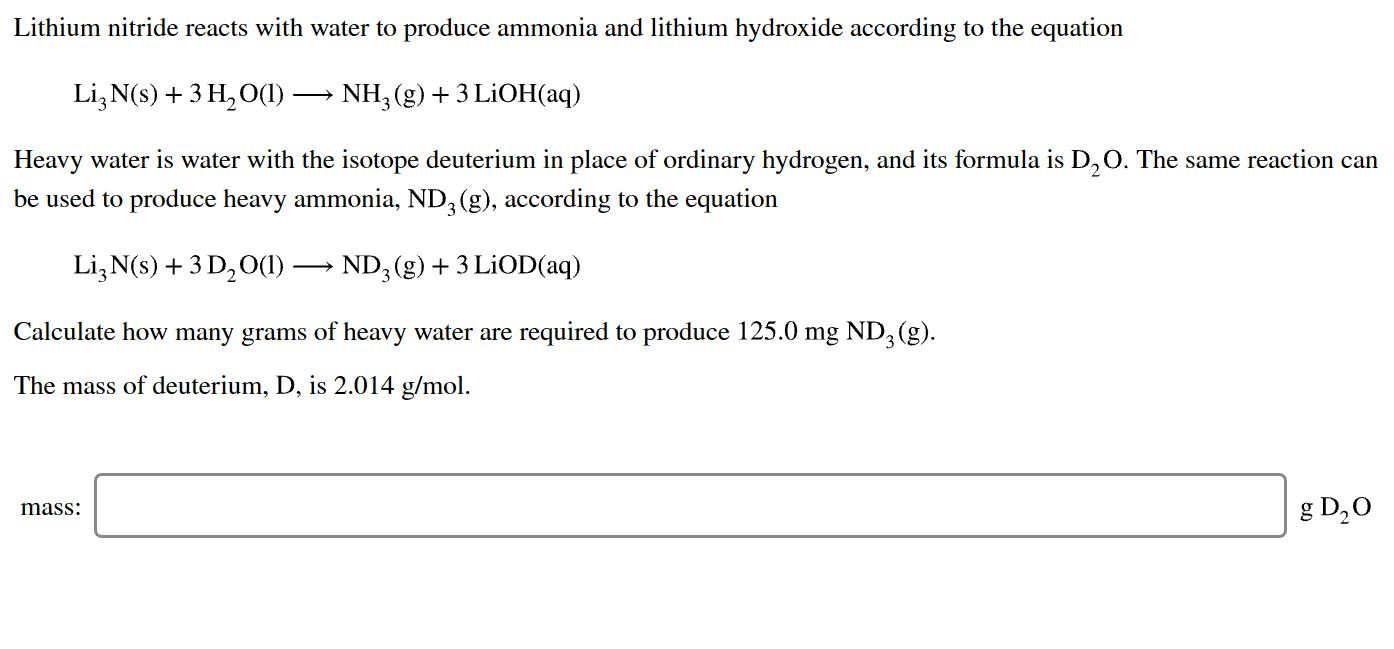# Lithium nitride reacts with water to produce ammonia and lithium hydroxide according to the equationLi3 N(s)3 H2 O()NH3 (g)3 LiOH(aq)Heavy water is water with the isotope deuterium in place of ordinary hydrogen, and its formula is D,O. The same reaction canbe used to produce heavy ammonia, ND, (g), according to the equationLi3 N(s)+3 D20()-ND, (g)3 LiOD(aq)Calculate how many grams of heavy water are required to produce 125.0 mg ND3 (g)The mass of deuterium, D, is 2.014 g/molg D20mass:

Question
55 viewshelp_outlineImage TranscriptioncloseLithium nitride reacts with water to produce ammonia and lithium hydroxide according to the equation Li3 N(s)3 H2 O() NH3 (g)3 LiOH(aq) Heavy water is water with the isotope deuterium in place of ordinary hydrogen, and its formula is D,O. The same reaction can be used to produce heavy ammonia, ND, (g), according to the equation Li3 N(s)+3 D20()-ND, (g)3 LiOD(aq) Calculate how many grams of heavy water are required to produce 125.0 mg ND3 (g) The mass of deuterium, D, is 2.014 g/mol g D20 mass: fullscreen
check_circle

Step 1

The number of moles for a given chemical species refers to the ratio of mass of the species to that of its molar mass. The formula is shown below:

Step 2

The term limiting reagent for a given reaction can be defined as the reactant which is totally consumed in a given chemical reaction when the reaction is completed. The quantity of products that can be formed in a given reaction gets limited by this reagent.

Step 3

The given chemical reacti...

### Want to see the full answer?

See Solution

#### Want to see this answer and more?

Solutions are written by subject experts who are available 24/7. Questions are typically answered within 1 hour.*

See Solution
*Response times may vary by subject and question.
Tagged in

### Physical Chemistry